Скачать презентацию Approximate NN queries on Streams with Guaranteed Error performance

da93f0c5d404543c29dabd095ec7d3e2.ppt

• Количество слайдов: 10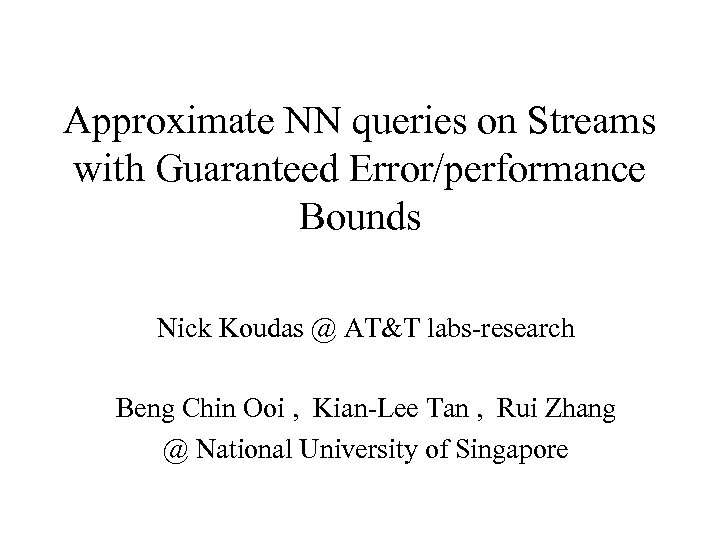Approximate NN queries on Streams with Guaranteed Error/performance Bounds Nick Koudas @ AT&T labs-research Beng Chin Ooi , Kian-Lee Tan , Rui Zhang @ National University of Singapore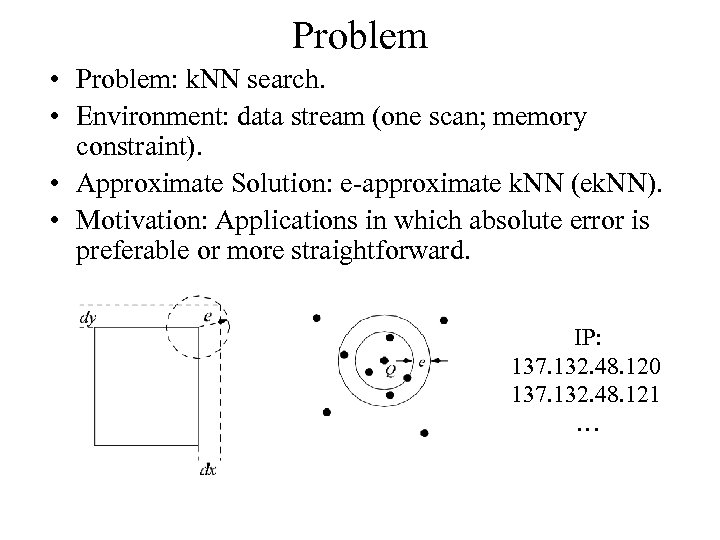Problem • Problem: k. NN search. • Environment: data stream (one scan; memory constraint). • Approximate Solution: e-approximate k. NN (ek. NN). • Motivation: Applications in which absolute error is preferable or more straightforward. IP: 137. 132. 48. 120 137. 132. 48. 121 …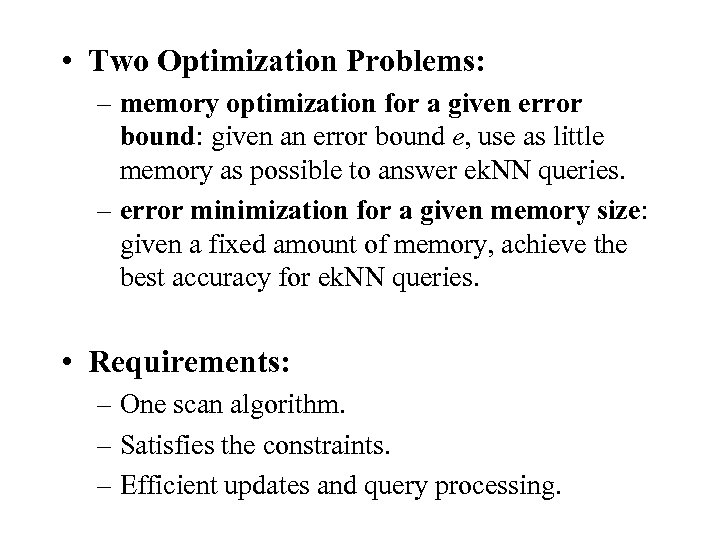• Two Optimization Problems: – memory optimization for a given error bound: given an error bound e, use as little memory as possible to answer ek. NN queries. – error minimization for a given memory size: given a fixed amount of memory, achieve the best accuracy for ek. NN queries. • Requirements: – One scan algorithm. – Satisfies the constraints. – Efficient updates and query processing.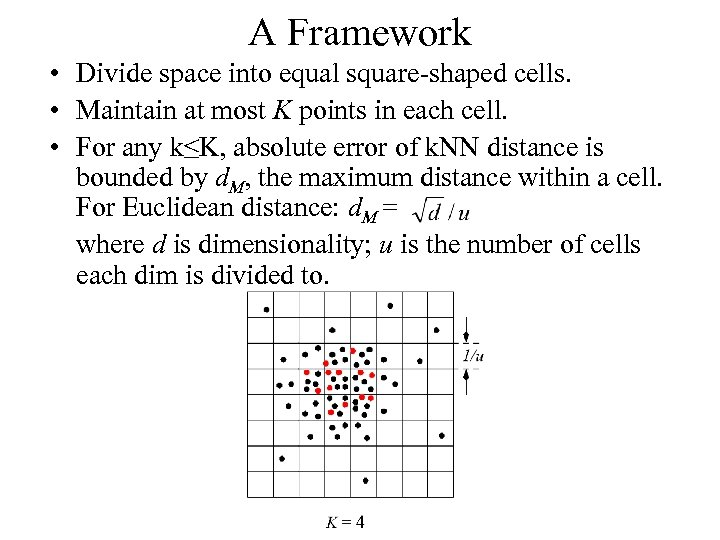A Framework • Divide space into equal square-shaped cells. • Maintain at most K points in each cell. • For any k≤K, absolute error of k. NN distance is bounded by d. M, the maximum distance within a cell. For Euclidean distance: d. M = where d is dimensionality; u is the number of cells each dim is divided to.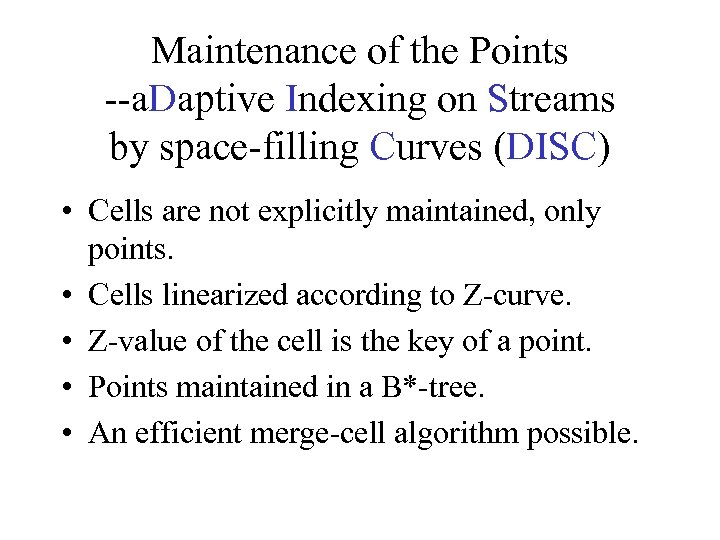Maintenance of the Points --a. Daptive Indexing on Streams by space-filling Curves (DISC) • Cells are not explicitly maintained, only points. • Cells linearized according to Z-curve. • Z-value of the cell is the key of a point. • Points maintained in a B*-tree. • An efficient merge-cell algorithm possible.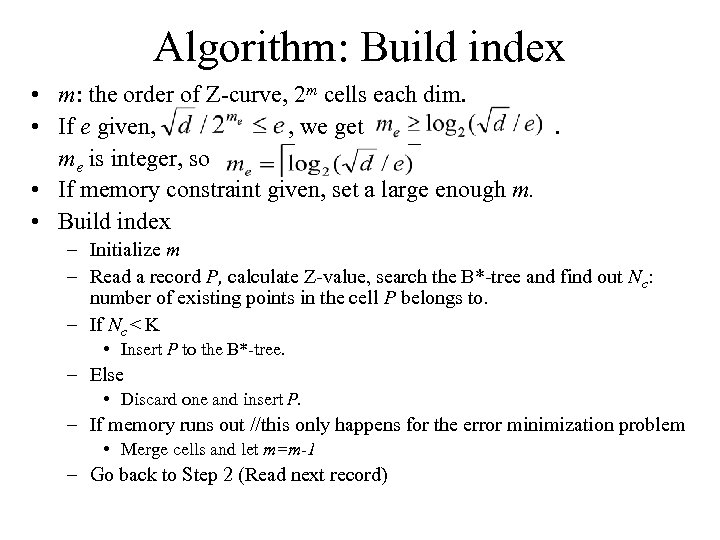Algorithm: Build index • m: the order of Z-curve, 2 m cells each dim. • If e given, , we get. me is integer, so • If memory constraint given, set a large enough m. • Build index – Initialize m – Read a record P, calculate Z-value, search the B*-tree and find out Nc: number of existing points in the cell P belongs to. – If Nc < K • Insert P to the B*-tree. – Else • Discard one and insert P. – If memory runs out //this only happens for the error minimization problem • Merge cells and let m=m-1 – Go back to Step 2 (Read next record)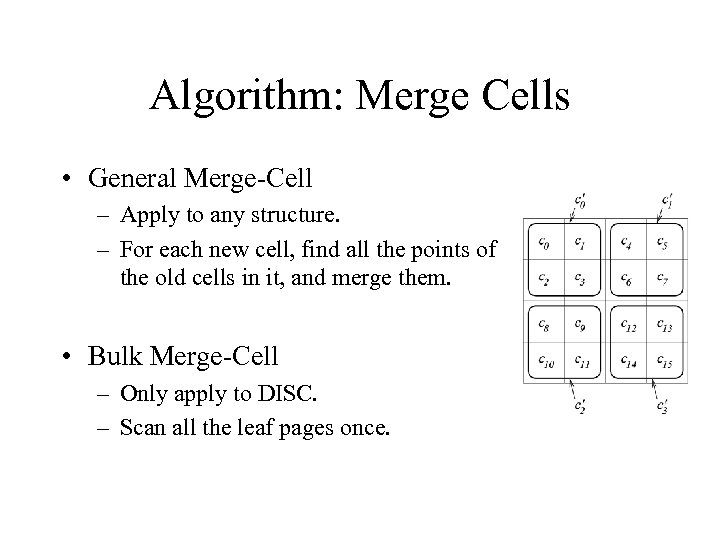Algorithm: Merge Cells • General Merge-Cell – Apply to any structure. – For each new cell, find all the points of the old cells in it, and merge them. • Bulk Merge-Cell – Only apply to DISC. – Scan all the leaf pages once.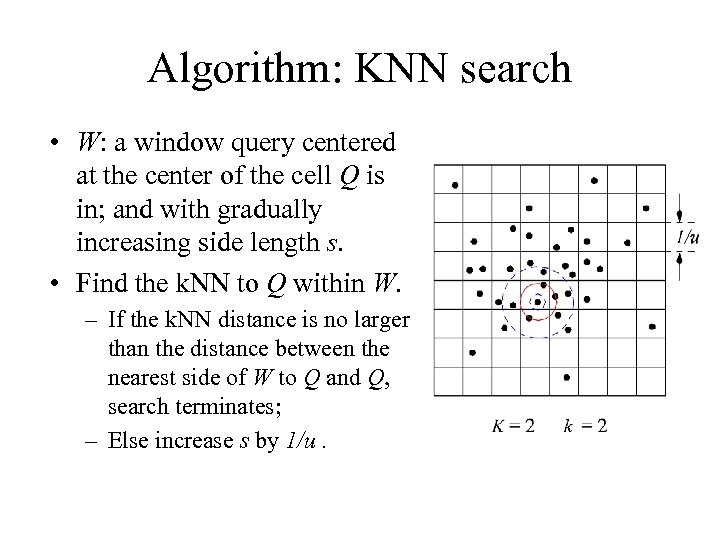Algorithm: KNN search • W: a window query centered at the center of the cell Q is in; and with gradually increasing side length s. • Find the k. NN to Q within W. – If the k. NN distance is no larger than the distance between the nearest side of W to Q and Q, search terminates; – Else increase s by 1/u.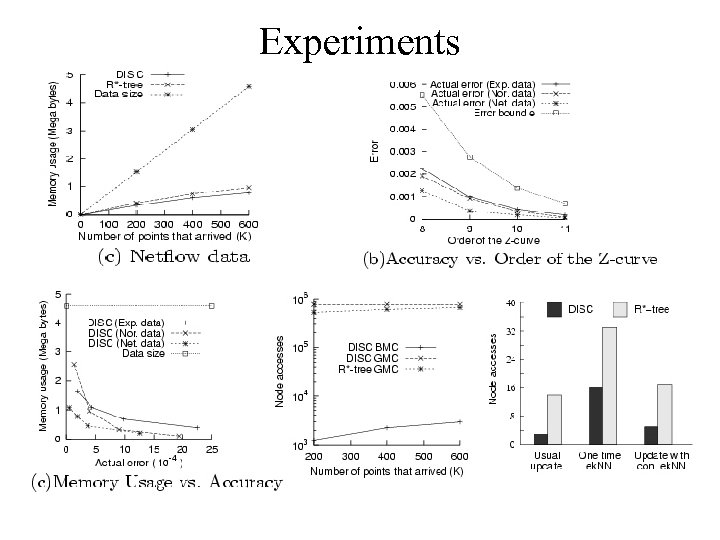ExperimentsQuestions ?